עברית Tell a Friend

# Research Areas

Name Research fieldProf. Ron Adin Algebraic combinatorics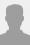Prof. Gideon Amir

Probability theory – with an emphasis on random walks, interacting particle systems and random graphs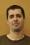Prof. Gil Ariel

Applied and computational mathematics: dynamical systems with multiple time scales, mathematicalDr. Baruch Barzel

Complex systems and non-linear dynamics, stochastic processes, networks, statistical physics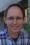Dr. Jonathan Beck Representation Theory, Quantum GroupsProf. Alexei Belov Ring theory, Semigroup theory,Polynomial automorphisms, Quantization,Symbolical dynamic combinatorial geometry and its mechanical applications, Elementary mathematics and Mathematical education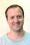Dr. Shimon Brooks Ergodic Theory, Spectral Theory, Quantum Chaos.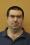Prof. Reuven Cohen

Complex networks, graph theory, probability, complexity, discrete geometry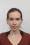Dr. Naomi Feldheim

Probability and Analysis, in particular: Gaussian functions, large deviations, concentration of measure, discrepancy theoryDr. Simcha (Simi) Haber

Financial Mathematics,, Computational Finance, Probabilistic Combinatorics, Finite Model Theory, Graph Theory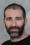Dr. Eyal Kaplan

Automorphic forms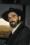Prof. Mikhail Katz Differential geometry, Riemannian geometry, low-dimensional topology, Riemann surfaces, mathematics education, history of mathematics, infinitesimals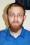Prof. Nathan Keller

Combinatorics – Discrete harmonic analysis and its applications to Combinatorics and related fields including Probability theory, Social choice theory etc. Cryptography – design and cryptanalysis of symmetric key cryptosystems.Prof. Yakov Krasnov Qualitative theory of partial differential equations, complex and hypercomplex analysis, numerical methods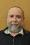Prof. Boris Kunyavski Algebraic geometry, algebraic groups (including computational problems and applications in coding theory and public-key cryptography)Dr. Emanuel (Menachem) Lazar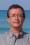Prof. Andrei Lerner Real analysis, harmonic analysis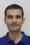Prof. Nir Lev Harmonic Analysis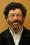Prof. Mordechay Levin Probabilistic diophantine approximation, applications of number theory to numerical analysis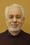Prof. Elijah Liflyand Harmonic analysis, approximation theory, functions of several complex variables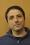Prof. Yoram Louzoun Mathematical biology, bioinformatics, graph theory, stochastic processes, machine learning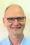Prof. Stuart Margolis Representation theory, semigroups, theory of automata, formal languages, computational algebra, combinatorial group theory, computational complexity theoryDr. Eli Matzri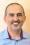Prof. Michael Megrel Groups of topological transformation and applications to functional analysisProf. Shahar Nevo Complex analysis: normal families, operator theory: rational matrix valued functions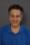Prof. Tahl Nowik Low dimensional topology, immersions of surfaces, plane and spherical curves, knot diagrams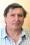Prof. Evgeny Plotkin Algebra, linear groupsDr. Shifra Reif

Representation theory,
Lie algebras and superalgebras,
Algebraic combinatorics,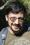Prof. Andre Reznikov Number theory, automorphic functions, representation theory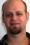Prof. Assaf Rinot

combinatorial set theory, especially around the combinatorics of singular cardinalsProf. Yuval Roichman Combinatorics, graph theory, representation theory, Coxeter groupsDr. Michael Schein Algebraic number theory, Galois representationsProf. Jeremy Schiff

Mathematical physics, differential equations, conformal field theory, integrable systems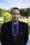Dr. Erez Sheiner

יסודות המבנה של האלגברה הסופרטרופיתProf. Leonid Shuster Differential equations, differential operators, difference operators, asymptotical problems for differential equations and operators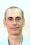Prof. Boris Solomyak

Fractal Geometry, Ergodic TheoryProf. Boaz Tsaban Pure mathematics: Applications of infinite combinatorics and other set theoretic methods to real analysis and general topology.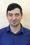Prof. Uzi Vishne

Ring theory, Division Algebras